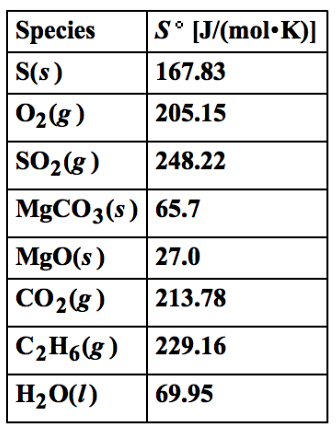Chemistry Practice Problems Entropy Practice Problems Solution: Using the data in the table, calculate the standar...

🤓 Based on our data, we think this question is relevant for Professor Delgado's class at FIU.

# Solution: Using the data in the table, calculate the standard entropy changes (J/mol•K) for the following reactions at 25 °C.S(s) + O2(g) → SO2(g)MgCO3(s) → MgO(s) + CO2(g)2 C2H6(g) + 7O2(g) → 4 CO2(g) + 6 H2O(l)

###### Problem

Using the data in the table, calculate the standard entropy changes (J/mol•K) for the following reactions at 25 °C.

S(s) + O2(g) → SO2(g)

MgCO3(s) → MgO(s) + CO2(g)

2 C2H6(g) + 7O2(g) → 4 CO2(g) + 6 H2O(l)View Complete Written Solution

Entropy

Entropy

#### Q. The ΔHvapo of a certain compound is 13.08 kJ mol-1 and its ΔSvapo is 67.74 J mol-1 K-1.What is the normal boiling point of this compound?

Solved • Fri Mar 30 2018 13:24:39 GMT-0400 (EDT)

Entropy

#### Q. ΔS is positive for the reaction _________________.a. 2NO(g) + O 2(g) → 2NO2(g)b. 2N2(g) + 2H2(g) → 2NH3(g)c. C3H8(g) + 5O2(g) → 3CO2(g) + 4H2O(g) d. M...

Solved • Wed Mar 28 2018 10:51:34 GMT-0400 (EDT)

Entropy

#### Q. Using the following data, calculate ΔSfus and ΔSvap for HBr.Determine the entropy change when 4.80 mol of HBr (f) freezes at atmospheric pressure.

Solved • Wed Mar 28 2018 10:08:33 GMT-0400 (EDT)

Entropy

#### Q. CO(NH2)2(aq) + H2O(l) → CO2(g) +2NH3(g) ΔS° = +354.8 J/mol*KGiven the data below, calculate the ΔS° f for UreaSubstance S° (J/molK)H2O (l) 69.96CO2 (g...

Solved • Fri Mar 23 2018 16:46:49 GMT-0400 (EDT)